# Equivalent circuit model example 1: gain equalizer

Click here to go to our main page on S-parameter model fitting

New for June 2022. In this example we will show how an equivalent circuit model is fit to the S-parameters of a broadband equalizer. The data we chose to fit is for a linear, positive-slope device from Dielectric Labs, now part of Knowles. This gain equalizer can be used for counter-act gain slope in 2-18 GHz systems, with a slope of nominally 3dB. You can google the part number shown in the schematic below if you want the data sheet.  The part is made to be mounted directly to a gap in a fifty-ohm trace on circuit card.

Below is a block that contains measured data. In this case, the measured data is from a manufacturer; in other cases, the data might not be measured at all. For example, data could be data from a 3D EM simulation.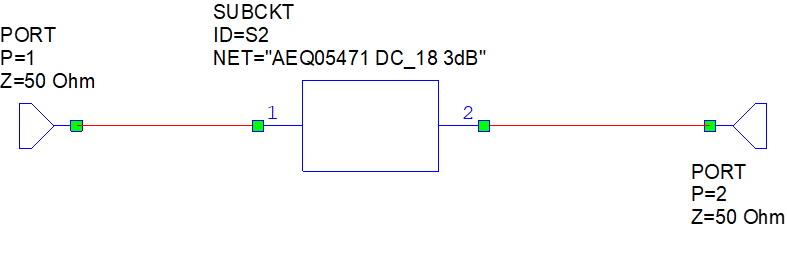The next block is an equivalent circuit we came up with for this type of equalizer. This is probably 95% of the magic of achieving a good fit. You can expect to spend some time playing with circuits that might not fit well, just keep optimizing, and deleting features that have very little effect on the outcome. Our final model is a simple, parallel LC network in series with the transmission line. Note that the design has no shunt resistive elements, so that it cannot possibly be matched to 50 ohms down at "DC". That is helpful when the equalizer designer raises the question, "what is the cost target for this part" and business development replies, "cheap as dirt".

The image below shows the equivalent circuit along with some starting values that we assigned to make a poor fit.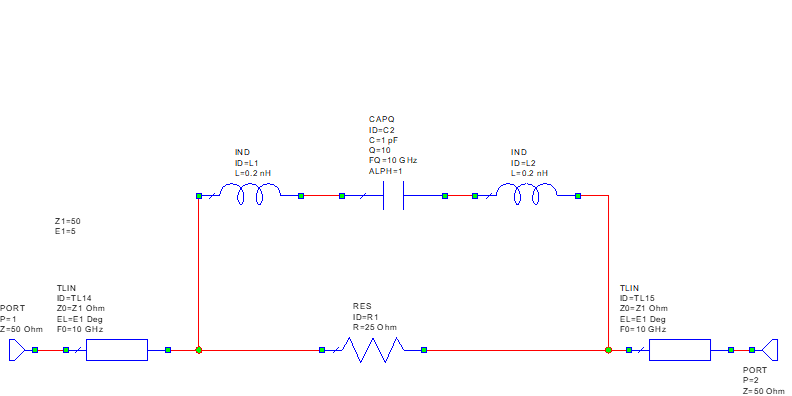Here is the start of the model fit.  We plot the magnitude of the errors... which means the maximum error for a passive network is a value of 2 (from one side of the Smith chart to the direct-opposite side). An error of 0.25, in technical terms, means your model fit sucks.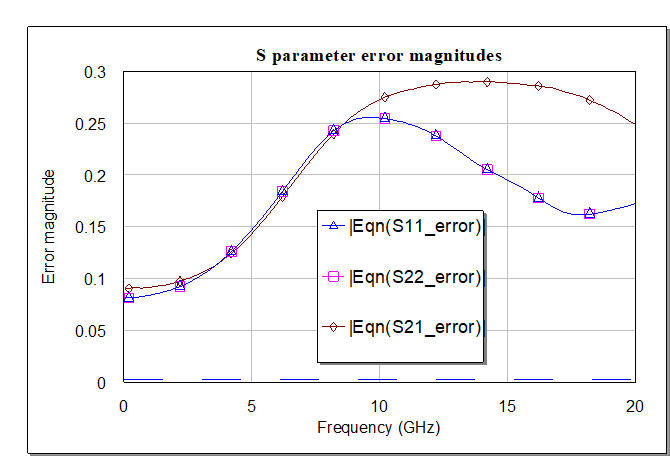Now it's time for an official Microwaves101 video! Click play, below, to watch the random optimizer's awesome powers...

Why do we use the random method instead of gradient or something fancier? Random usually never misses the ultimate minimum value of the error function, it works well enough so why use anything else? The random optimizer is like a dive bar, while the other offerings are what you might call "fern bars" that would cause your father to laugh at your expense if he knew you went into them.

Below are the errors when the optimizer has been run until stagnation. The errors are quite small as you will see when we plot S-parameters of model and measurement.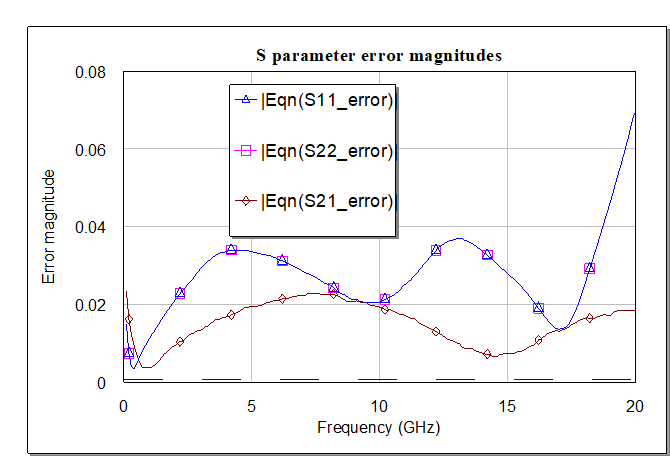Here are optimized values for the equivalent circuit. Note that inductors that connect the capacitor are slightly different, which was necessary as S11 and S22 are slightly different. The Q of the capacitor is really poor (4.6) as one plate of the cap is probably the thin-film resistor!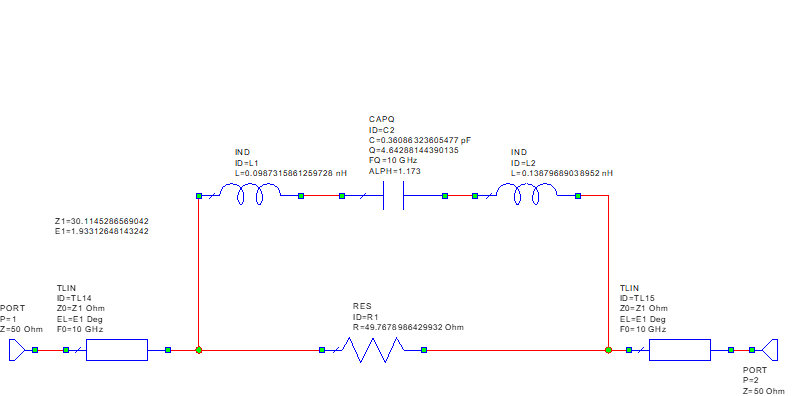Now let's look at S-parameter fit. Here are S11 measured and modelled on the same chart. Here is the input reflection coefficient, it is spot on.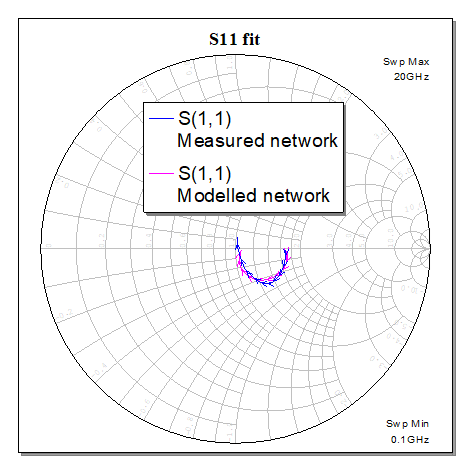Here is that parameter plotted in dB. You see we are slightly missing the exact dip near 18 GHz, so you could spend some more time on the model if you like.  We're done here.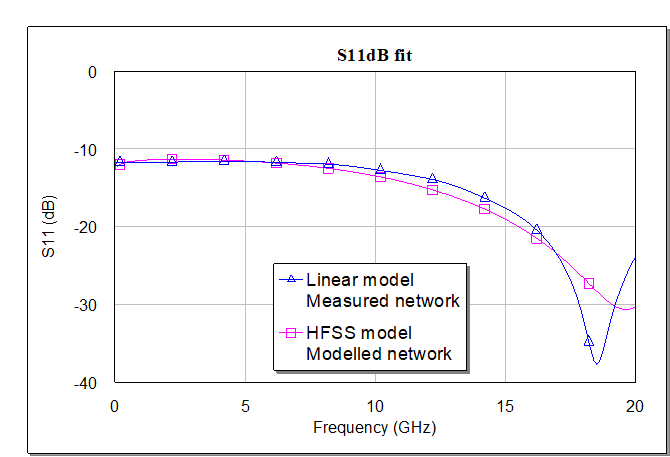Below is the output reflection coefficient fit. Note that the measured data is slightly different for S11 and S22, which is why we allowed our network to optimize assymetrically (the inductors are slightly different values).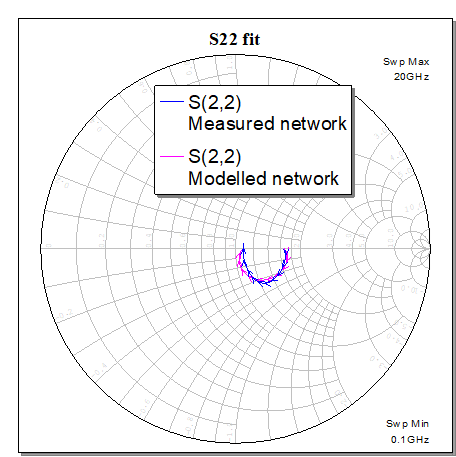Perhaps the most important fit is the transmission coefficient, which is what makes it a gain equalizer.  Over most of the and we are within 0.1 dB of the truth.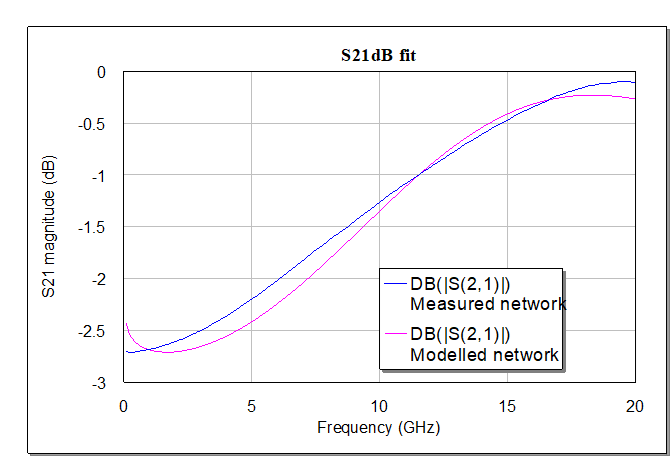Would you like a copy of the Microwave Office file that generated these results?  Just ask!  Eventually we will put it into our download area.